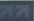Back to cetaka's profile on different computer, have to save this here lolPosted on: December 13, 2007, at 02:41:59am(yet another alternate and admittedly less elegant solution for b2 that I found yesterday while avoiding studying for exams, but it's still pretty awesome because it uses taylor's formula hehe! :D) we're given a continuous function f:[0,1]->R such that int_0^1 f(x) dx = 0. so define g by g(x)= int_0^a f(t) dt. then clearly g(0)=g(1)=0 and g'(x) is just f(x). now we use taylor's formula to arrive at the solution. normally we would use that to say something like "f(x)=f(0)+f'(0)*(x-0)+.5*f"(c)*(x-0)^2 " for some c on the interval, but here we need to consider the formula about x with respect to 0 and 1. thus, g(0)=g(x)+g'(x)*(0-x)+.5*g"(a)*(0-x)^2 and g(1)=g(x)+g'(x)*.5*g"(b)*(1-x)^2, where a and b are of course in [0,1]. subtracting, you get: g'(x) - .5*g"(a) + .5*[g"(b)-2x*g"(b)+g"(b)] = 0. But wait! since g'(x)=f(x), g"(x) = f'(x), which is continuous on a closed interval and thus bounded by some constant K. now we are getting somewhere...... 0 \$/leq\$ f(x) - xK + K/2, or -f(x) \$/leq\$ K(1/2-x). aha! now we integrate both sides from 0 to a to get: /int_0^a -f(x) \$/leq\$ (K/2)*(a-a^2). since a is in [0,1] we are done. so yeah, kinda lengthy compared to the other ones we came up with, but extra methods never hurt anybody :P